Date

Only dead fish go with the flow.

As I promised in the last article, today we’re finally going to do a deep dive into the topic of scopes.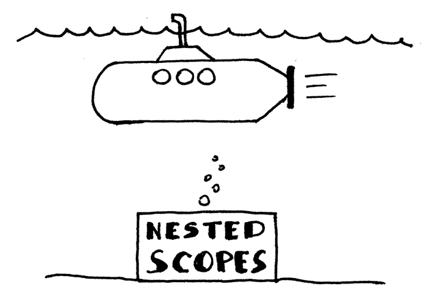This is what we’re going to learn today:

• We’re going to learn about scopes, why they are useful, and how to implement them in code with symbol tables.
• We’re going to learn about nested scopes and how chained scoped symbol tables are used to implement nested scopes.
• We’re going to learn how to parse procedure declarations with formal parameters and how to represent a procedure symbol in code.
• We’re going to learn how to extend our semantic analyzer to do semantic checks in the presence of nested scopes.
• We’re going to learn more about name resolution and how the semantic analyzer resolves names to their declarations when a program has nested scopes.
• We’re going to learn how to build a scope tree.
• We’re also going to learn how to write our very own source-to-source compiler today! We will see later in the article how relevant it is to our discussion of scopes.

Let’s get started! Or should I say, let’s dive in!

### Scopes and scoped symbol tables

What is a scope? A scope is a textual region of a program where a name can be used. Let’s take a look at the following sample program, for example:

```program Main;
var x, y: integer;
begin
x := x + y;
end.
```

In Pascal, the PROGRAM keyword (case insensitive, by the way) introduces a new scope which is commonly called a global scope, so the program above has one global scope and the declared variables x and y are visible and accessible in the whole program. In the case above, the textual region starts with the keyword program and ends with the keyword end and a dot. In that textual region both names x and y can be used, so the scope of those variables (variable declarations) is the whole program: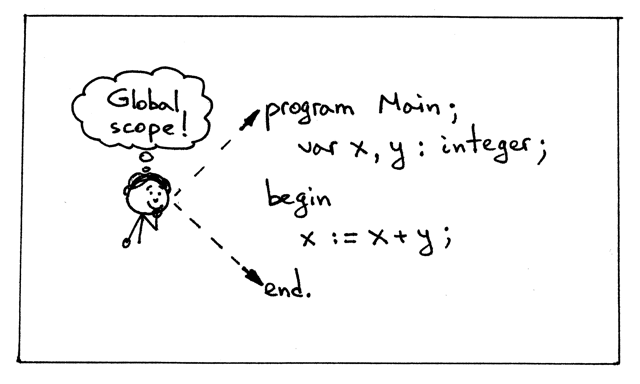When you look at the source code above and specifically at the expression x := x + y, you intuitively know that it should compile (or get interpreted) without a problem, because the scope of the variables x and y in the expression is the global scope and the variable references x and y in the expression x := x + y resolve to the declared integer variables x and y. If you’ve programmed before in any mainstream programming language, there shouldn’t be any surprises here.

When we talk about the scope of a variable, we actually talk about the scope of its declaration: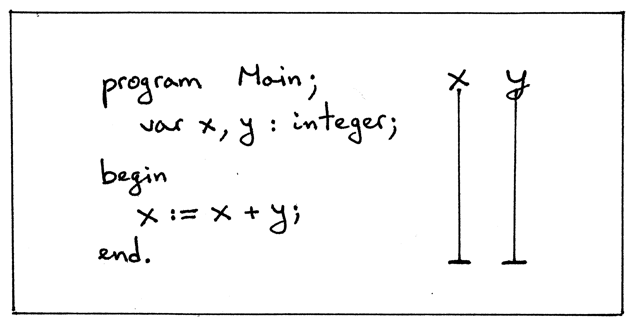In the picture above, the vertical lines show the scope of the declared variables, the textual region where the declared names x and y can be used, that is, the text area where they are visible. And as you can see, the scope of x and y is the whole program, as shown by the vertical lines.

Pascal programs are said to be lexically scoped (or statically scoped) because you can look at the source code, and without even executing the program, determine purely based on the textual rules which names (references) resolve or refer to which declarations. In Pascal, for example, lexical keywords like program and end demarcate the textual boundaries of a scope: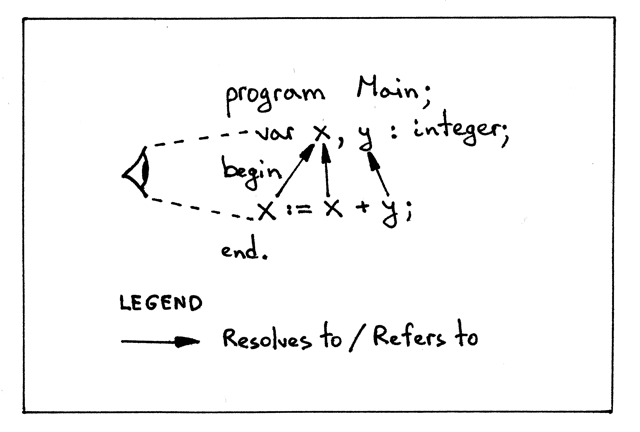Why are scopes useful?

• Every scope creates an isolated name space, which means that variables declared in a scope cannot be accessed from outside of it.
• You can re-use the same name in different scopes and know exactly, just by looking at the program source code, what declaration the name refers to at every point in the program.
• In a nested scope you can re-declare a variable with the same name as in the outer scope, thus effectively hiding the outer declaration, which gives you control over access to different variables from the outer scope.

In addition to the global scope, Pascal supports nested procedures, and every procedure declaration introduces a new scope, which means that Pascal supports nested scopes.

When we talk about nested scopes, it’s convenient to talk about scope levels to show their nesting relationships. It’s also convenient to refer to scopes by name. We’ll use both scope levels and scope names when we start our discussion of nested scopes.

Let’s take a look at the following sample program and subscript every name in the program to make it clear:

1. At what level each variable (symbol) is declared
2. To which declaration and at what level a variable name refers to: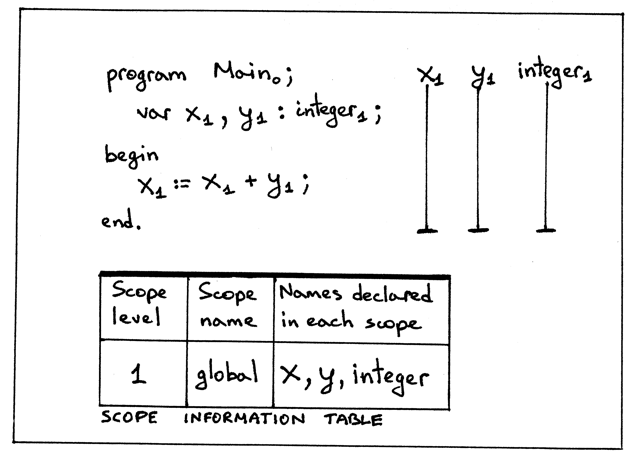From the picture above we can see several things:

• We have a single scope, the global scope, introduced by the PROGRAM keyword
• Global scope is at level 1
• Variables (symbols) x and y are declared at level 1 (the global scope).
• integer built-in type is also declared at level 1
• The program name Main has a subscript 0. Why is the program’s name at level zero, you might wonder? This is to make it clear that the program’s name is not in the global scope and it’s in some other outer scope, that has level zero.
• The scope of the variables x and y is the whole program, as shown by the vertical lines
• The scope information table shows for every level in the program the corresponding scope level, scope name, and names declared in the scope. The purpose of the table is to summarize and visually show different information about scopes in a program.

How do we implement the concept of a scope in code? To represent a scope in code, we’ll need a scoped symbol table. We already know about symbol tables, but what is a scoped symbol table? A scoped symbol table is basically a symbol table with a few modifications, as you’ll see shortly.

From now on, we’ll use the word scope both to mean the concept of a scope as well as to refer to the scoped symbol table, which is an implementation of the scope in code.

Even though in our code a scope is represented by an instance of the ScopedSymbolTable class, we’ll use the variable named scope throughout the code for convenience. So when you see a variable scope in the code of our interpreter, you should know that it actually refers to a scoped symbol table.

Okay, let’s enhance our SymbolTable class by renaming it to ScopedSymbolTable class, adding two new fields scope_level and scope_name, and updating the scoped symbol table’s constructor. And at the same time, let’s update the __str__ method to print additional information, namely the scope_level and scope_name. Here is a new version of the symbol table, the ScopedSymbolTable:

```class ScopedSymbolTable(object):
def __init__(self, scope_name, scope_level):
self._symbols = OrderedDict()
self.scope_name = scope_name
self.scope_level = scope_level
self._init_builtins()

def _init_builtins(self):
self.insert(BuiltinTypeSymbol('INTEGER'))
self.insert(BuiltinTypeSymbol('REAL'))

def __str__(self):
h1 = 'SCOPE (SCOPED SYMBOL TABLE)'
lines = ['\n', h1, '=' * len(h1)]
('Scope name', self.scope_name),
('Scope level', self.scope_level),
):
h2 = 'Scope (Scoped symbol table) contents'
lines.extend([h2, '-' * len(h2)])
lines.extend(
('%7s: %r' % (key, value))
for key, value in self._symbols.items()
)
lines.append('\n')
s = '\n'.join(lines)
return s

__repr__ = __str__

def insert(self, symbol):
print('Insert: %s' % symbol.name)
self._symbols[symbol.name] = symbol

def lookup(self, name):
print('Lookup: %s' % name)
symbol = self._symbols.get(name)
# 'symbol' is either an instance of the Symbol class or None
return symbol
```

Let’s also update the semantic analyzer’s code to use the variable scope instead of symtab, and remove the semantic check that was checking source programs for duplicate identifiers from the visit_VarDecl method to reduce the noise in the program output.

Here is a piece of code that shows how our semantic analyzer instantiates the ScopedSymbolTable class:

```class SemanticAnalyzer(NodeVisitor):
def __init__(self):
self.scope = ScopedSymbolTable(scope_name='global', scope_level=1)

...
```

You can find all the changes in the file scope01.py. Download the file, run it on the command line, and inspect the output. Here is what I got:

```\$ python scope01.py
Insert: INTEGER
Insert: REAL
Lookup: INTEGER
Insert: x
Lookup: INTEGER
Insert: y
Lookup: x
Lookup: y
Lookup: x

SCOPE (SCOPED SYMBOL TABLE)
===========================
Scope name     : global
Scope level    : 1
Scope (Scoped symbol table) contents
------------------------------------
INTEGER: <BuiltinTypeSymbol(name='INTEGER')>
REAL: <BuiltinTypeSymbol(name='REAL')>
x: <VarSymbol(name='x', type='INTEGER')>
y: <VarSymbol(name='y', type='INTEGER')>
```

Most of the output should look very familiar to you.

Now that you know about the concept of scope and how to implement the scope in code by using a scoped symbol table, it’s time we talked about nested scopes and more dramatic modifications to the scoped symbol table than just adding two simple fields.

### Procedure declarations with formal parameters

Let’s take a look at a sample program in the file nestedscopes02.pas that contains a procedure declaration:

```program Main;
var x, y: real;

procedure Alpha(a : integer);
var y : integer;
begin
x := a + x + y;
end;

begin { Main }

end.  { Main }
```

The first thing that we notice here is that we have a procedure with a parameter, and we haven’t learned how to handle that yet. Let’s fill that gap by making a quick detour and learning how to handle formal procedure parameters before continuing with scopes.*

*ASIDE: Formal parameters are parameters that show up in the declaration of a procedure. Arguments (also called actual parameters) are different variables and expressions passed to a procedure in a particular procedure call.

Here is a list of changes we need to make to support procedure declarations with parameters:

1. Add the Param AST node

```class Param(AST):
def __init__(self, var_node, type_node):
self.var_node = var_node
self.type_node = type_node
```
2. Update the ProcedureDecl node’s constructor to take an additional argument: params

```class ProcedureDecl(AST):
def __init__(self, proc_name, params, block_node):
self.proc_name = proc_name
self.params = params  # a list of Param nodes
self.block_node = block_node
```
3. Update the declarations rule to reflect changes in the procedure declaration sub-rule

```def declarations(self):
"""declarations : (VAR (variable_declaration SEMI)+)*
| (PROCEDURE ID (LPAREN formal_parameter_list RPAREN)? SEMI block SEMI)*
| empty
"""
```
4. Add the formal_parameter_list rule and method

```def formal_parameter_list(self):
""" formal_parameter_list : formal_parameters
| formal_parameters SEMI formal_parameter_list
"""
```
5. Add the formal_parameters rule and method

```def formal_parameters(self):
""" formal_parameters : ID (COMMA ID)* COLON type_spec """
param_nodes = []
```

With the addition of the above methods and rules our parser will be able to parse procedure declarations like these (I’m not showing the body of declared procedures for brevity):

```procedure Foo;

procedure Foo(a : INTEGER);

procedure Foo(a, b : INTEGER);

procedure Foo(a, b : INTEGER; c : REAL);
```

Let’s generate an AST for our sample program. Download genastdot.py and run the following command on the command line:

```\$ python genastdot.py nestedscopes02.pas > ast.dot && dot -Tpng -o ast.png ast.dot
```

Here is a picture of the generated AST: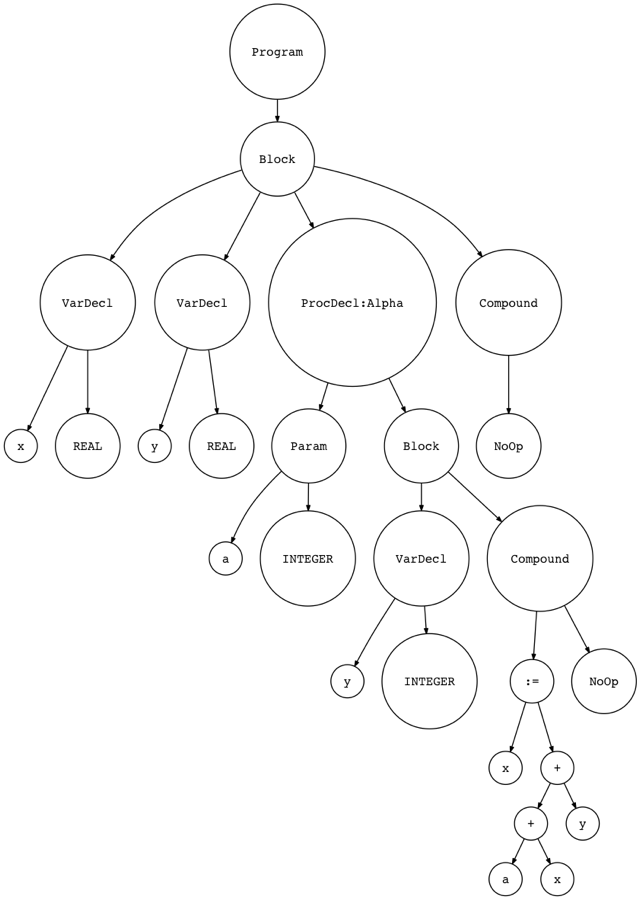You can see now that the ProcedureDecl node in the picture has the Param node as its child.

You can find the complete changes in the spi.py file. Spend some time and study the changes. You’ve done similar changes before; they should be pretty easy to understand and you should be able to implement them by yourself.

### Procedure symbols

While we’re on the topic of procedure declarations, let’s also talk about procedure symbols.

As with variable declarations, and built-in type declarations, there is a separate category of symbols for procedures. Let’s create a separate symbol class for procedure symbols:

```class ProcedureSymbol(Symbol):
def __init__(self, name, params=None):
super(ProcedureSymbol, self).__init__(name)
# a list of formal parameters
self.params = params if params is not None else []

def __str__(self):
return '<{class_name}(name={name}, parameters={params})>'.format(
class_name=self.__class__.__name__,
name=self.name,
params=self.params,
)

__repr__ = __str__
```

Procedure symbols have a name (it’s a procedure’s name), their category is procedure (it’s encoded in the class name), and the type is None because in Pascal procedures don’t return anything.

Procedure symbols also carry additional information about procedure declarations, namely they contain information about the procedure’s formal parameters as you can see in the code above.

With the addition of procedure symbols, our new symbol hierarchy looks like this: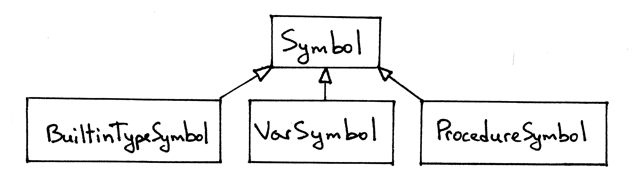### Nested scopes

After that quick detour let’s get back to our program and the discussion of nested scopes:

```program Main;
var x, y: real;

procedure Alpha(a : integer);
var y : integer;
begin
x := a + x + y;
end;

begin { Main }

end.  { Main }
```

Things are actually getting more interesting here. By declaring a new procedure, we introduce a new scope, and this scope is nested within the global scope introduced by the PROGRAM statement, so this is a case where we have nested scopes in a Pascal program.

The scope of a procedure is the whole body of the procedure. The beginning of the procedure scope is marked by the PROCEDURE keyword and the end is marked by the END keyword and a semicolon.

Let’s subscript names in the program and show some additional information: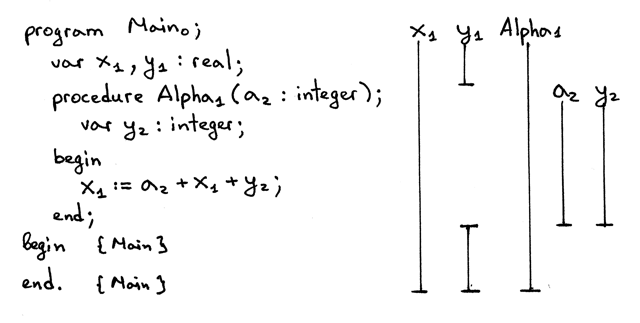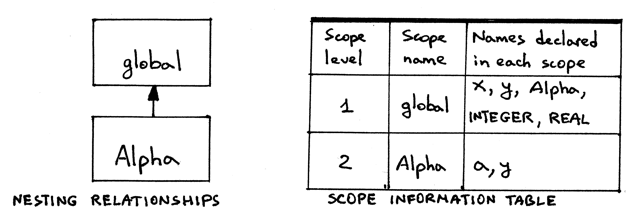Some observations from the picture above:

• This Pascal program has two scope levels: level 1 and level 2
• The nesting relationships diagram visually shows that the scope Alpha is nested within the global scope, hence there are two levels: the global scope at level 1, and the Alpha scope at level 2.
• The scope level of the procedure declaration Alpha is one less than the level of the variables declared inside the procedure Alpha. You can see that the scope level of the procedure declaration Alpha is 1 and the scope level of the variables a and y inside the procedure is 2.
• The variable declaration of y inside Alpha hides the declaration of y in the global scope. You can see the hole in the vertical bar for y1 (by the way, 1 is a subscript, it’s not part of the variable name, the variable name is just y) and you can see that the scope of the y2 variable declaration is the Alpha procedure’s whole body.
• The scope information table, as you are already aware, shows scope levels, scope names for those levels, and respective names declared in those scopes (at those levels).
• In the picture, you can also see that I omitted showing the scope of the integer and real types (except in the scope information table) because they are always declared at scope level 1, the global scope, so I won’t be subscripting the integer and real types anymore to save visual space, but you will see the types again and again in the contents of the scoped symbol table representing the global scope.

The next step is to discuss implementation details.

First, let’s focus on variable and procedure declarations. Then, we’ll discuss variable references and how name resolution works in the presence of nested scopes.

For our discussion, we’ll use a stripped down version of the program. The following version does not have variable references: it only has variable and procedure declarations:

```program Main;
var x, y: real;

procedure Alpha(a : integer);
var y : integer;
begin

end;

begin { Main }

end.  { Main }
```

You already know how to represent a scope in code with a scoped symbol table. Now we have two scopes: the global scope and the scope introduced by the procedure Alpha. Following our approach we should now have two scoped symbol tables: one for the global scope and one for the Alpha scope. How do we implement that in code? We’ll extend the semantic analyzer to create a separate scoped symbol table for every scope instead of just for the global scope. The scope construction will happen, as usual, when walking the AST.

First, we need to decide where in the semantic analyzer we’re going to create our scoped symbol tables. Recall that PROGRAM and PROCEDURE keywords introduce new scope. In AST, the corresponding nodes are Program and ProcedureDecl. So we’re going to update our visit_Program method and add the visit_ProcedureDecl method to create scoped symbol tables. Let’s start with the visit_Program method:

```def visit_Program(self, node):
print('ENTER scope: global')
global_scope = ScopedSymbolTable(
scope_name='global',
scope_level=1,
)
self.current_scope = global_scope

# visit subtree
self.visit(node.block)

print(global_scope)
print('LEAVE scope: global')
```

The method has quite a few changes:

1. When visiting the node in AST, we first print what scope we’re entering, in this case global.
2. We create a separate scoped symbol table to represent the global scope. When we construct an instance of ScopedSymbolTable, we explicitly pass the scope name and scope level arguments to the class constructor.
3. We assign the newly created scope to the instance variable current_scope. Other visitor methods that insert and look up symbols in scoped symbol tables will use the current_scope.
4. We visit a subtree (block). This is the old part.
5. Before leaving the global scope we print the contents of the global scope (scoped symbol table)
6. We also print the message that we’re leaving the global scope

Now let’s add the visit_ProcedureDecl method. Here is the complete source code for it:

```def visit_ProcedureDecl(self, node):
proc_name = node.proc_name
proc_symbol = ProcedureSymbol(proc_name)
self.current_scope.insert(proc_symbol)

print('ENTER scope: %s' %  proc_name)
# Scope for parameters and local variables
procedure_scope = ScopedSymbolTable(
scope_name=proc_name,
scope_level=2,
)
self.current_scope = procedure_scope

# Insert parameters into the procedure scope
for param in node.params:
param_type = self.current_scope.lookup(param.type_node.value)
param_name = param.var_node.value
var_symbol = VarSymbol(param_name, param_type)
self.current_scope.insert(var_symbol)
proc_symbol.params.append(var_symbol)

self.visit(node.block_node)

print(procedure_scope)
print('LEAVE scope: %s' %  proc_name)
```

Let’s go over the contents of the method:

1. The first thing that the method does is create a procedure symbol and insert it into the current scope, which is the global scope for our sample program.
2. Then the method prints the message about entering the procedure scope.
3. Then we create a new scope for the procedure’s parameters and variable declarations.
4. We assign the procedure scope to the self.current_scope variable indicating that this is our current scope and all symbol operations (insert and lookup) will use the current scope.
5. Then we handle procedure formal parameters by inserting them into the current scope and adding them to the procedure symbol.
6. Then we visit the rest of the AST subtree - the body of the procedure.
7. And, finally, we print the message about leaving the scope before leaving the node and moving to another AST node, if any.

Now, what we need to do is update other semantic analyzer visitor methods to use self.current_scope when inserting and looking up symbols. Let’s do that:

```def visit_VarDecl(self, node):
type_name = node.type_node.value
type_symbol = self.current_scope.lookup(type_name)

# We have all the information we need to create a variable symbol.
# Create the symbol and insert it into the symbol table.
var_name = node.var_node.value
var_symbol = VarSymbol(var_name, type_symbol)

self.current_scope.insert(var_symbol)

def visit_Var(self, node):
var_name = node.value
var_symbol = self.current_scope.lookup(var_name)
if var_symbol is None:
raise Exception(
)
```

Both the visit_VarDecl and visit_Var will now use the current_scope to insert and/or look up symbols. Specifically, for our sample program, the current_scope can point either to the global scope or the Alpha scope.

We also need to update the semantic analyzer and set the current_scope to None in the constructor:

```class SemanticAnalyzer(NodeVisitor):
def __init__(self):
self.current_scope = None
```

Clone the GitHub repository for the article, run scope02.py (it has all the changes we just discussed), inspect the output, and make sure you understand why every line is generated:

```\$ python scope02.py
ENTER scope: global
Insert: INTEGER
Insert: REAL
Lookup: REAL
Insert: x
Lookup: REAL
Insert: y
Insert: Alpha
ENTER scope: Alpha
Insert: INTEGER
Insert: REAL
Lookup: INTEGER
Insert: a
Lookup: INTEGER
Insert: y

SCOPE (SCOPED SYMBOL TABLE)
===========================
Scope name     : Alpha
Scope level    : 2
Scope (Scoped symbol table) contents
------------------------------------
INTEGER: <BuiltinTypeSymbol(name='INTEGER')>
REAL: <BuiltinTypeSymbol(name='REAL')>
a: <VarSymbol(name='a', type='INTEGER')>
y: <VarSymbol(name='y', type='INTEGER')>

LEAVE scope: Alpha

SCOPE (SCOPED SYMBOL TABLE)
===========================
Scope name     : global
Scope level    : 1
Scope (Scoped symbol table) contents
------------------------------------
INTEGER: <BuiltinTypeSymbol(name='INTEGER')>
REAL: <BuiltinTypeSymbol(name='REAL')>
x: <VarSymbol(name='x', type='REAL')>
y: <VarSymbol(name='y', type='REAL')>
Alpha: <ProcedureSymbol(name=Alpha, parameters=[<VarSymbol(name='a', type='INTEGER')>])>

LEAVE scope: global
```

Some things about the output above that I think are worth mentioning:

1. You can see that the two lines Insert: INTEGER and Insert: REAL are repeated twice in the output and the keys INTEGER and REAL are present in both scopes (scoped symbol tables): global and Alpha. The reason is that we create a separate scoped symbol table for every scope and the table initializes the built-in type symbols every time we create its instance. We’ll change it later when we discuss nesting relationships and how they are expressed in code.
2. See how the line Insert: Alpha is printed before the line ENTER scope: Alpha. This is just a reminder that a name of a procedure is declared at a level that is one less than the level of the variables declared in the procedure itself.
3. You can see by inspecting the printed contents of the scoped symbol tables above what declarations they contain. See, for example, that global scope has the Alpha symbol in it.
4. From the contents of the global scope you can also see that the procedure symbol for the Alpha procedure also contains the procedure’s formal parameters.

After we run the program, our scopes in memory would look something like this, just two separate scoped symbol tables: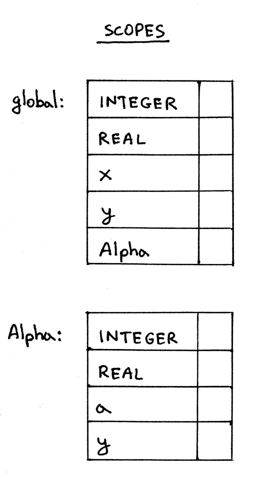### Scope tree: Chaining scoped symbol tables

Okay, now every scope is represented by a separate scoped symbol table, but how do we represent the nesting relationship between the global scope and the scope Alpha as we showed in the nesting relationship diagram before? In other words, how do we express in code that the scope Alpha is nested within the global scope? The answer is chaining the tables together.

We’ll chain the scoped symbol tables together by creating a link between them. In a way it’ll be like a tree (we’ll call it a scope tree), just an unusual one, because in this tree a child will be pointing to a parent, and not the other way around. Let’s take a look the following scope tree: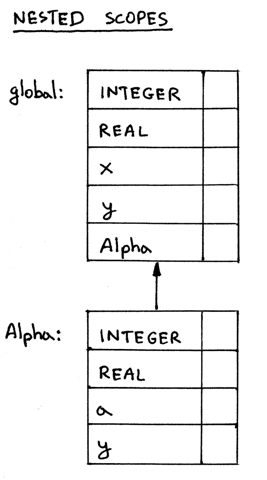In the scope tree above you can see that the scope Alpha is linked to the global scope by pointing to it. To put it differently, the scope Alpha is pointing to its enclosing scope, which is the global scope. It all means that the scope Alpha is nested within the global scope.

How do we implement scope chaining/linking? There are two steps:

1. We need to update the ScopedSymbolTable class and add a variable enclosing_scope that will hold a pointer to the scope’s enclosing scope. This will be the link between scopes in the picture above.
2. We need to update the visit_Program and visit_ProcedureDecl methods to create an actual link to the scope’s enclosing scope using the updated version of the ScopedSymbolTable class.

Let’s start with updating the ScopedSymbolTable class and adding the enclosing_scope field. Let’s also update the __init__ and __str__ methods. The __init__ method will be modified to accept a new parameter, enclosing_scope, with the default value set to None. The __str__ method will be updated to output the name of the enclosing scope. Here is the complete source code of the updated ScopedSymbolTable class:

```class ScopedSymbolTable(object):
def __init__(self, scope_name, scope_level, enclosing_scope=None):
self._symbols = OrderedDict()
self.scope_name = scope_name
self.scope_level = scope_level
self.enclosing_scope = enclosing_scope
self._init_builtins()

def _init_builtins(self):
self.insert(BuiltinTypeSymbol('INTEGER'))
self.insert(BuiltinTypeSymbol('REAL'))

def __str__(self):
h1 = 'SCOPE (SCOPED SYMBOL TABLE)'
lines = ['\n', h1, '=' * len(h1)]
('Scope name', self.scope_name),
('Scope level', self.scope_level),
('Enclosing scope',
self.enclosing_scope.scope_name if self.enclosing_scope else None
)
):
h2 = 'Scope (Scoped symbol table) contents'
lines.extend([h2, '-' * len(h2)])
lines.extend(
('%7s: %r' % (key, value))
for key, value in self._symbols.items()
)
lines.append('\n')
s = '\n'.join(lines)
return s

__repr__ = __str__

def insert(self, symbol):
print('Insert: %s' % symbol.name)
self._symbols[symbol.name] = symbol

def lookup(self, name):
print('Lookup: %s' % name)
symbol = self._symbols.get(name)
# 'symbol' is either an instance of the Symbol class or None
return symbol
```

Now let’s switch our attention to the visit_Program method:

```def visit_Program(self, node):
print('ENTER scope: global')
global_scope = ScopedSymbolTable(
scope_name='global',
scope_level=1,
enclosing_scope=self.current_scope, # None
)
self.current_scope = global_scope

# visit subtree
self.visit(node.block)

print(global_scope)

self.current_scope = self.current_scope.enclosing_scope
print('LEAVE scope: global')
```

There are a couple of things here worth mentioning and repeating:

1. We explicitly pass the self.current_scope as the enclosing_scope argument when creating a scope
2. We assign the newly created global scope to the variable self.current_scope
3. We restore the variable self.current_scope to its previous value right before leaving the Program node. It’s important to restore the value of the current_scope after we’ve finished processing the node, otherwise the scope tree construction will be broken when we have more than two scopes in our program. We’ll see why shortly.

And, finally, let’s update the visit_ProcedureDecl method:

```def visit_ProcedureDecl(self, node):
proc_name = node.proc_name
proc_symbol = ProcedureSymbol(proc_name)
self.current_scope.insert(proc_symbol)

print('ENTER scope: %s' %  proc_name)
# Scope for parameters and local variables
procedure_scope = ScopedSymbolTable(
scope_name=proc_name,
scope_level=self.current_scope.scope_level + 1,
enclosing_scope=self.current_scope
)
self.current_scope = procedure_scope

# Insert parameters into the procedure scope
for param in node.params:
param_type = self.current_scope.lookup(param.type_node.value)
param_name = param.var_node.value
var_symbol = VarSymbol(param_name, param_type)
self.current_scope.insert(var_symbol)
proc_symbol.params.append(var_symbol)

self.visit(node.block_node)

print(procedure_scope)

self.current_scope = self.current_scope.enclosing_scope
print('LEAVE scope: %s' %  proc_name)
```

Again, the main changes compared to the version in scope02.py are:

1. We explicitly pass the self.current_scope as an enclosing_scope argument when creating a scope.
2. We no longer hard code the scope level of a procedure declaration because we can calculate the level automatically based on the scope level of the procedure’s enclosing scope: it’s the enclosing scope’s level plus one.
3. We restore the value of the self.current_scope to its previous value (for our sample program the previous value would be the global scope) right before leaving the ProcedureDecl node.

Okay, let’s see what the contents of the scoped symbol tables look like with the above changes. You can find all the changes in scope03a.py. Our sample program is:

```program Main;
var x, y: real;

procedure Alpha(a : integer);
var y : integer;
begin

end;

begin { Main }

end.  { Main }
```

Run scope03a.py on the command line and inspect the output:

```\$ python scope03a.py
ENTER scope: global
Insert: INTEGER
Insert: REAL
Lookup: REAL
Insert: x
Lookup: REAL
Insert: y
Insert: Alpha
ENTER scope: Alpha
Insert: INTEGER
Insert: REAL
Lookup: INTEGER
Insert: a
Lookup: INTEGER
Insert: y

SCOPE (SCOPED SYMBOL TABLE)
===========================
Scope name     : Alpha
Scope level    : 2
Enclosing scope: global
Scope (Scoped symbol table) contents
------------------------------------
INTEGER: <BuiltinTypeSymbol(name='INTEGER')>
REAL: <BuiltinTypeSymbol(name='REAL')>
a: <VarSymbol(name='a', type='INTEGER')>
y: <VarSymbol(name='y', type='INTEGER')>

LEAVE scope: Alpha

SCOPE (SCOPED SYMBOL TABLE)
===========================
Scope name     : global
Scope level    : 1
Enclosing scope: None
Scope (Scoped symbol table) contents
------------------------------------
INTEGER: <BuiltinTypeSymbol(name='INTEGER')>
REAL: <BuiltinTypeSymbol(name='REAL')>
x: <VarSymbol(name='x', type='REAL')>
y: <VarSymbol(name='y', type='REAL')>
Alpha: <ProcedureSymbol(name=Alpha, parameters=[<VarSymbol(name='a', type='INTEGER')>])>

LEAVE scope: global
```

You can see in the output above that the global scope doesn’t have an enclosing scope and, the Alpha‘s enclosing scope is the global scope, which is what we would expect, because the Alpha scope is nested within the global scope.

Now, as promised, let’s consider why it is important to set and restore the value of the self.current_scope variable. Let’s take a look at the following program, where we have two procedure declarations in the global scope:

```program Main;
var x, y : real;

procedure AlphaA(a : integer);
var y : integer;
begin { AlphaA }

end;  { AlphaA }

procedure AlphaB(a : integer);
var b : integer;
begin { AlphaB }

end;  { AlphaB }

begin { Main }

end.  { Main }
```

The nesting relationship diagram for the sample program looks like this: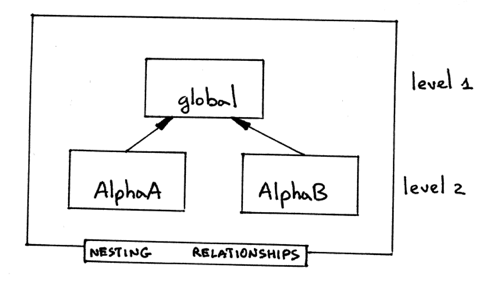An AST for the program (I left only the nodes that are relevant to this example) is something like this: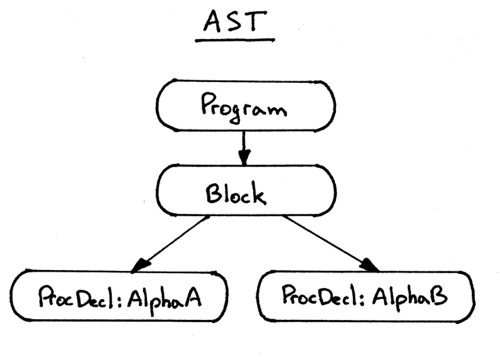If we don’t restore the current scope when we leave the Program and ProcedureDecl nodes what is going to happen? Let’s see.

The way our semantic analyzer walks the tree is depth first, left-to-right, so it will traverse the ProcedureDecl node for AlphaA first and then it will visit the ProcedureDecl node for AlphaB. The problem here is that if we don’t restore the self.current_scope before leaving AlphaA the self.current_scope will be left pointing to AlphaA instead of the global scope and, as a result, the semantic analyzer will create the scope AlphaB at level 3, as if it was nested within the scope AlphaA, which is, of course, incorrect.

To see the broken behavior when the current scope is not being restored before leaving Program and/or ProcedureDecl nodes, download and run the scope03b.py on the command line:

```\$ python scope03b.py
ENTER scope: global
Insert: INTEGER
Insert: REAL
Lookup: REAL
Insert: x
Lookup: REAL
Insert: y
Insert: AlphaA
ENTER scope: AlphaA
Insert: INTEGER
Insert: REAL
Lookup: INTEGER
Insert: a
Lookup: INTEGER
Insert: y

SCOPE (SCOPED SYMBOL TABLE)
===========================
Scope name     : AlphaA
Scope level    : 2
Enclosing scope: global
Scope (Scoped symbol table) contents
------------------------------------
INTEGER: <BuiltinTypeSymbol(name='INTEGER')>
REAL: <BuiltinTypeSymbol(name='REAL')>
a: <VarSymbol(name='a', type='INTEGER')>
y: <VarSymbol(name='y', type='INTEGER')>

LEAVE scope: AlphaA
Insert: AlphaB
ENTER scope: AlphaB
Insert: INTEGER
Insert: REAL
Lookup: INTEGER
Insert: a
Lookup: INTEGER
Insert: b

SCOPE (SCOPED SYMBOL TABLE)
===========================
Scope name     : AlphaB
Scope level    : 3
Enclosing scope: AlphaA
Scope (Scoped symbol table) contents
------------------------------------
INTEGER: <BuiltinTypeSymbol(name='INTEGER')>
REAL: <BuiltinTypeSymbol(name='REAL')>
a: <VarSymbol(name='a', type='INTEGER')>
b: <VarSymbol(name='b', type='INTEGER')>

LEAVE scope: AlphaB

SCOPE (SCOPED SYMBOL TABLE)
===========================
Scope name     : global
Scope level    : 1
Enclosing scope: None
Scope (Scoped symbol table) contents
------------------------------------
INTEGER: <BuiltinTypeSymbol(name='INTEGER')>
REAL: <BuiltinTypeSymbol(name='REAL')>
x: <VarSymbol(name='x', type='REAL')>
y: <VarSymbol(name='y', type='REAL')>
AlphaA: <ProcedureSymbol(name=AlphaA, parameters=[<VarSymbol(name='a', type='INTEGER')>])>

LEAVE scope: global
```

As you can see, scope tree construction in our semantic analyzer is completely broken in the presence of more than two scopes:

1. Instead of two scope levels as shown in the nesting relationships diagram, we have three levels
2. The global scope contents doesn’t have AlphaB in it, only AlphaA.

To construct a scope tree correctly, we need to follow a really simple procedure:

1. When we ENTER a Program or ProcedureDecl node, we create a new scope and assign it to the self.current_scope.
2. When we are about to LEAVE the Program or ProcedureDecl node, we restore the value of the self.current_scope.

You can think of the self.current_scope as a stack pointer and a scope tree as a collection of stacks:

1. When you visit a Program or ProcedureDecl node, you push a new scope on the stack and adjust the stack pointer self.current_scope to point to the top of stack, which is now the most recently pushed scope.
2. When you are about to leave the node, you pop the scope off the stack and you also adjust the stack pointer to point to the previous scope on the stack, which is now the new top of stack.

To see the correct behavior in the presence of multiple scopes, download and run scope03c.py on the command line. Study the output. Make sure you understand what is going on:

```\$ python scope03c.py
ENTER scope: global
Insert: INTEGER
Insert: REAL
Lookup: REAL
Insert: x
Lookup: REAL
Insert: y
Insert: AlphaA
ENTER scope: AlphaA
Insert: INTEGER
Insert: REAL
Lookup: INTEGER
Insert: a
Lookup: INTEGER
Insert: y

SCOPE (SCOPED SYMBOL TABLE)
===========================
Scope name     : AlphaA
Scope level    : 2
Enclosing scope: global
Scope (Scoped symbol table) contents
------------------------------------
INTEGER: <BuiltinTypeSymbol(name='INTEGER')>
REAL: <BuiltinTypeSymbol(name='REAL')>
a: <VarSymbol(name='a', type='INTEGER')>
y: <VarSymbol(name='y', type='INTEGER')>

LEAVE scope: AlphaA
Insert: AlphaB
ENTER scope: AlphaB
Insert: INTEGER
Insert: REAL
Lookup: INTEGER
Insert: a
Lookup: INTEGER
Insert: b

SCOPE (SCOPED SYMBOL TABLE)
===========================
Scope name     : AlphaB
Scope level    : 2
Enclosing scope: global
Scope (Scoped symbol table) contents
------------------------------------
INTEGER: <BuiltinTypeSymbol(name='INTEGER')>
REAL: <BuiltinTypeSymbol(name='REAL')>
a: <VarSymbol(name='a', type='INTEGER')>
b: <VarSymbol(name='b', type='INTEGER')>

LEAVE scope: AlphaB

SCOPE (SCOPED SYMBOL TABLE)
===========================
Scope name     : global
Scope level    : 1
Enclosing scope: None
Scope (Scoped symbol table) contents
------------------------------------
INTEGER: <BuiltinTypeSymbol(name='INTEGER')>
REAL: <BuiltinTypeSymbol(name='REAL')>
x: <VarSymbol(name='x', type='REAL')>
y: <VarSymbol(name='y', type='REAL')>
AlphaA: <ProcedureSymbol(name=AlphaA, parameters=[<VarSymbol(name='a', type='INTEGER')>])>
AlphaB: <ProcedureSymbol(name=AlphaB, parameters=[<VarSymbol(name='a', type='INTEGER')>])>

LEAVE scope: global
```

This is how our scoped symbol tables look like after we’ve run scope03c.py and correctly constructed the scope tree: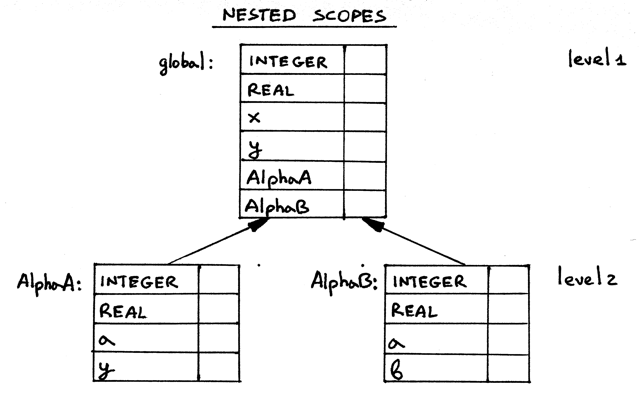Again, as I’ve mentioned above, you can think of the scope tree above as a collection of scope stacks.

Now let’s continue and talk about how name resolution works when we have nested scopes.

### Nested scopes and name resolution

Our focus before was on variable and procedure declarations. Let’s add variable references to the mix.

Here is a sample program with some variable references in it:

```program Main;
var x, y: real;

procedure Alpha(a : integer);
var y : integer;
begin
x := a + x + y;
end;

begin { Main }

end.  { Main }
```

Or visually with some additional information:Let’s turn our attention to the assignment statement x := a + x + y; Here it is with subscripts: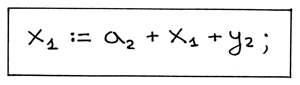We see that x resolves to a declaration at level 1, a resolves to a declaration at level 2 and y also resolves to a declaration at level 2. How does that resolution work? Let’s see how.

Lexically (statically) scoped languages like Pascal follow the most closely nested scope rule when it comes to name resolution. It means that, in every scope, a name refers to its lexically closest declaration. For our assignment statement, let’s go over every variable reference and see how the rule works in practice:

1. Because our semantic analyzer visits the right-hand side of the assignment first, we’ll start with the variable reference a from the arithmetic expression a + x + y. We begin our search for a‘s declaration in the lexically closest scope, which is the Alpha scope. The Alpha scope contains variable declarations in the Alpha procedure including the procedure’s formal parameters. We find the declaration of a in the Alpha scope: it’s the formal parameter a of the Alpha procedure - a variable symbol that has type integer. We usually do the search by scanning the source code with our eyes when resolving names (remember, a2 is not the name of a variable, 2 is the subscript here, the variable name is a):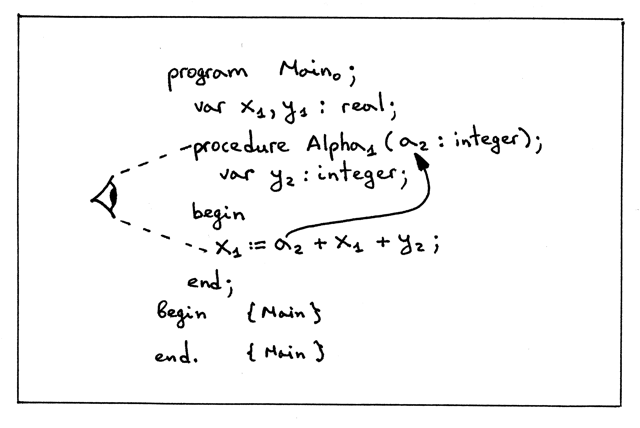2. Now onto the variable reference x from the arithmetic expression a + x + y. Again, first we search for the declaration of x in the lexically closest scope. The lexically closest scope is the Alpha scope at level 2. The scope contains declarations in the Alpha procedure including the procedure’s formal parameters. We don’t find x at this scope level (in the Alpha scope), so we go up the chain to the global scope and continue our search there. Our search succeeds because the global scope has a variable symbol with the name x in it: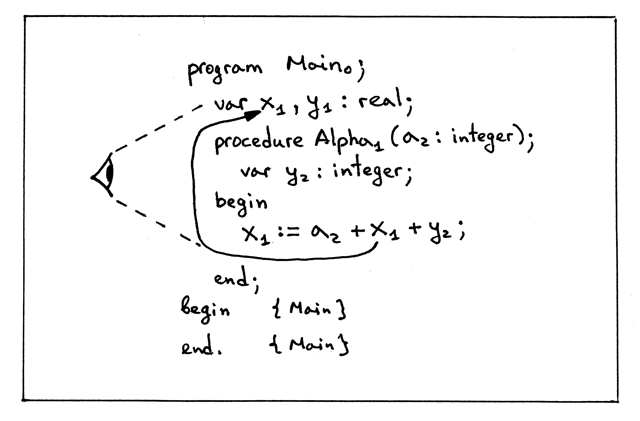3. Now, let’s look at the variable reference y from the arithmetic expression a + x + y. We find its declaration in the lexically closest scope, which is the Alpha scope. In the Alpha scope the variable y has type integer (if there weren’t a declaration for y in the Alpha scope we would scan the text and find y in the outer/global scope and it would have real type in that case):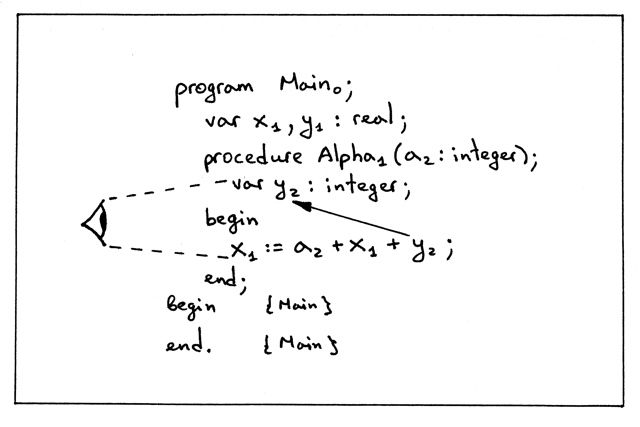4. And, finally, the variable x from the left hand side of the assignment statement x := a + x + y; It resolves to the same declaration as the variable reference x in the arithmetic expression on the right-hand side: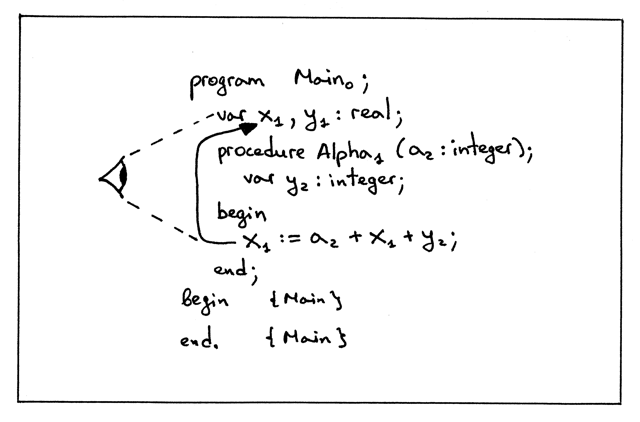How do we implement that behavior of looking in the current scope, and then looking in the enclosing scope, and so on until we either find the symbol we’re looking for or we’ve reached the top of the scope tree and there are no more scopes left? We simply need to extend the lookup method in the ScopedSymbolTable class to continue its search up the chain in the scope tree:

```def lookup(self, name):
print('Lookup: %s. (Scope name: %s)' % (name, self.scope_name))
# 'symbol' is either an instance of the Symbol class or None
symbol = self._symbols.get(name)

if symbol is not None:
return symbol

# recursively go up the chain and lookup the name
if self.enclosing_scope is not None:
return self.enclosing_scope.lookup(name)
```

The way the updated lookup method works:

1. Search for a symbol by name in the current scope. If the symbol is found, then return it.
2. If the symbol is not found, recursively traverse the tree and search for the symbol in the scopes up the chain. You don’t have to do the lookup recursively, you can rewrite it into an iterative form; the important part is to follow the link from a nested scope to its enclosing scope and search for the symbol there and up the tree until either the symbol is found or there are no more scopes left because you’ve reached the top of the scope tree.
3. The lookup method also prints the scope name, in parenthesis, where the lookup happens to make it clearer that lookup goes up the chain to search for a symbol, if it can’t find it in the current scope.

Let’s see what our semantic analyzer outputs for our sample program now that we’ve modified the way the lookup searches the scope tree for a symbol. Download scope04a.py and run it on the command line:

```\$ python scope04a.py
ENTER scope: global
Insert: INTEGER
Insert: REAL
Lookup: REAL. (Scope name: global)
Insert: x
Lookup: REAL. (Scope name: global)
Insert: y
Insert: Alpha
ENTER scope: Alpha
Lookup: INTEGER. (Scope name: Alpha)
Lookup: INTEGER. (Scope name: global)
Insert: a
Lookup: INTEGER. (Scope name: Alpha)
Lookup: INTEGER. (Scope name: global)
Insert: y
Lookup: a. (Scope name: Alpha)
Lookup: x. (Scope name: Alpha)
Lookup: x. (Scope name: global)
Lookup: y. (Scope name: Alpha)
Lookup: x. (Scope name: Alpha)
Lookup: x. (Scope name: global)

SCOPE (SCOPED SYMBOL TABLE)
===========================
Scope name     : Alpha
Scope level    : 2
Enclosing scope: global
Scope (Scoped symbol table) contents
------------------------------------
a: <VarSymbol(name='a', type='INTEGER')>
y: <VarSymbol(name='y', type='INTEGER')>

LEAVE scope: Alpha

SCOPE (SCOPED SYMBOL TABLE)
===========================
Scope name     : global
Scope level    : 1
Enclosing scope: None
Scope (Scoped symbol table) contents
------------------------------------
INTEGER: <BuiltinTypeSymbol(name='INTEGER')>
REAL: <BuiltinTypeSymbol(name='REAL')>
x: <VarSymbol(name='x', type='REAL')>
y: <VarSymbol(name='y', type='REAL')>
Alpha: <ProcedureSymbol(name=Alpha, parameters=[<VarSymbol(name='a', type='INTEGER')>])>

LEAVE scope: global
```

Inspect the output above and pay attention to the ENTER and Lookup messages. A couple of things worth mentioning here:

1. Notice how the semantic analyzer looks up the INTEGER built-in type symbol before inserting the variable symbol a. It searches INTEGER first in the current scope, Alpha, doesn’t find it, then goes up the tree all the way to the global scope, and finds the symbol there:

```ENTER scope: Alpha
Lookup: INTEGER. (Scope name: Alpha)
Lookup: INTEGER. (Scope name: global)
Insert: a
```
2. Notice also how the analyzer resolves variable references from the assignment statement x := a + x + y:

```Lookup: a. (Scope name: Alpha)
Lookup: x. (Scope name: Alpha)
Lookup: x. (Scope name: global)
Lookup: y. (Scope name: Alpha)
Lookup: x. (Scope name: Alpha)
Lookup: x. (Scope name: global)
```

The analyzer starts its search in the current scope and then goes up the tree all the way to the global scope.

Let’s also see what happens when a Pascal program has a variable reference that doesn’t resolve to a variable declaration as in the sample program below:

```program Main;
var x, y: real;

procedure Alpha(a : integer);
var y : integer;
begin
x := b + x + y; { ERROR here! }
end;

begin { Main }

end.  { Main }
```

```\$ python scope04b.py
ENTER scope: global
Insert: INTEGER
Insert: REAL
Lookup: REAL. (Scope name: global)
Insert: x
Lookup: REAL. (Scope name: global)
Insert: y
Insert: Alpha
ENTER scope: Alpha
Lookup: INTEGER. (Scope name: Alpha)
Lookup: INTEGER. (Scope name: global)
Insert: a
Lookup: INTEGER. (Scope name: Alpha)
Lookup: INTEGER. (Scope name: global)
Insert: y
Lookup: b. (Scope name: Alpha)
Lookup: b. (Scope name: global)
```

As you can see, the analyzer tried to resolve the variable reference b and searched for it in the Alpha scope first, then the global scope, and, not being able to find a symbol with the name b, it threw the semantic error.

Okay great, now we know how to write a semantic analyzer that can analyze a program for semantic errors when the program has nested scopes.

### Source-to-source compiler

Now, onto something completely different. Let’s write a source-to-source compiler! Why would we do it? Aren’t we talking about interpreters and nested scopes? Yes, we are, but let me explain why I think it might be a good idea to learn how to write a source-to-source compiler right now.

First, let’s talk about definitions. What is a source-to-source compiler? For the purpose of this article, let’s define a source-to-source compiler as a compiler that translates a program in some source language into a program in the same (or almost the same) source language.

So, if you write a translator that takes as an input a Pascal program and outputs a Pascal program, possibly modified, or enhanced, the translator in this case is called a source-to-source compiler.

A good example of a source-to-source compiler for us to study would be a compiler that takes a Pascal program as an input and outputs a Pascal-like program where every name is subscripted with a corresponding scope level, and, in addition to that, every variable reference also has a type indicator. So we want a source-to-source compiler that would take the following Pascal program:

```program Main;
var x, y: real;

procedure Alpha(a : integer);
var y : integer;
begin
x := a + x + y;
end;

begin { Main }

end.  { Main }
```

and turn it into the following Pascal-like program:

```program Main0;
var x1 : REAL;
var y1 : REAL;
procedure Alpha1(a2 : INTEGER);
var y2 : INTEGER;

begin
<x1:REAL> := <a2:INTEGER> + <x1:REAL> + <y2:INTEGER>;
end; {END OF Alpha}

begin

end. {END OF Main}
```

Here is the list of modifications our source-to-source compiler should make to an input Pascal program:

1. Every declaration should be printed on a separate line, so if we have multiple declarations in the input Pascal program, the compiled output should have each declaration on a separate line. We can see in the text above, for example, how the line var x, y : real; gets converted into multiple lines.
2. Every name should get subscripted with a number corresponding to the scope level of the respective declaration.
3. Every variable reference, in addition to being subscripted, should also be printed in the following form: <var_name_with_subscript:type>
4. The compiler should also add a comment at the end of every block in the form {END OF … }, where the ellipses will get substituted either with a program name or procedure name. That will help us identify the textual boundaries of procedures faster.

As you can see from the generated output above, this source-to-source compiler could be a useful tool for understanding how name resolution works, especially when a program has nested scopes, because the output generated by the compiler would allow us to quickly see to what declaration and in what scope a certain variable reference resolves to. This is good help when learning about symbols, nested scopes, and name resolution.

How can we implement a source-to-source compiler like that? We have actually covered all the necessary parts to do it. All we need to do now is extend our semantic analyzer a bit to generate the enhanced output. You can see the full source code of the compiler here. It is basically a semantic analyzer on drugs, modified to generate and return strings for certain AST nodes.

Download src2srccompiler.py, study it, and experiment with it by passing it different Pascal programs as an input.

For the following program, for example:

```program Main;
var x, y : real;
var z : integer;

procedure AlphaA(a : integer);
var y : integer;
begin { AlphaA }
x := a + x + y;
end;  { AlphaA }

procedure AlphaB(a : integer);
var b : integer;
begin { AlphaB }
end;  { AlphaB }

begin { Main }
end.  { Main }
```

The compiler generates the following output:

```\$ python src2srccompiler.py nestedscopes03.pas
program Main0;
var x1 : REAL;
var y1 : REAL;
var z1 : INTEGER;
procedure AlphaA1(a2 : INTEGER);
var y2 : INTEGER;

begin
<x1:REAL> := <a2:INTEGER> + <x1:REAL> + <y2:INTEGER>;
end; {END OF AlphaA}
procedure AlphaB1(a2 : INTEGER);
var b2 : INTEGER;

begin

end; {END OF AlphaB}

begin

end. {END OF Main}
```

Cool beans and congratulations, now you know how to write a basic source-to-source compiler!

Use it to further your understanding of nested scopes, name resolution, and what you can do when you have an AST and some extra information about the program in the form of symbol tables.

Now that we have a useful tool to subscript our programs for us, let’s take a look at a bigger example of nested scopes that you can find in nestedscopes04.pas:

```program Main;
var b, x, y : real;
var z : integer;

procedure AlphaA(a : integer);
var b : integer;

procedure Beta(c : integer);
var y : integer;

procedure Gamma(c : integer);
var x : integer;
begin { Gamma }
x := a + b + c + x + y + z;
end;  { Gamma }

begin { Beta }

end;  { Beta }

begin { AlphaA }

end;  { AlphaA }

procedure AlphaB(a : integer);
var c : real;
begin { AlphaB }
c := a + b;
end;  { AlphaB }

begin { Main }
end.  { Main }
```

Below you can see the declarations’ scopes, nesting relationships diagram, and scope information table: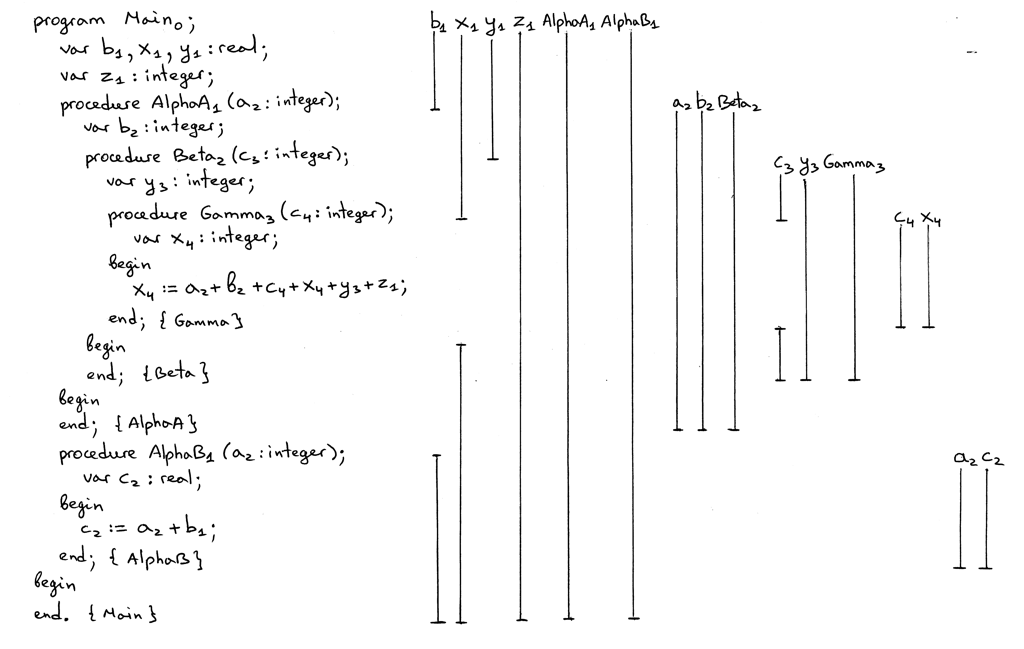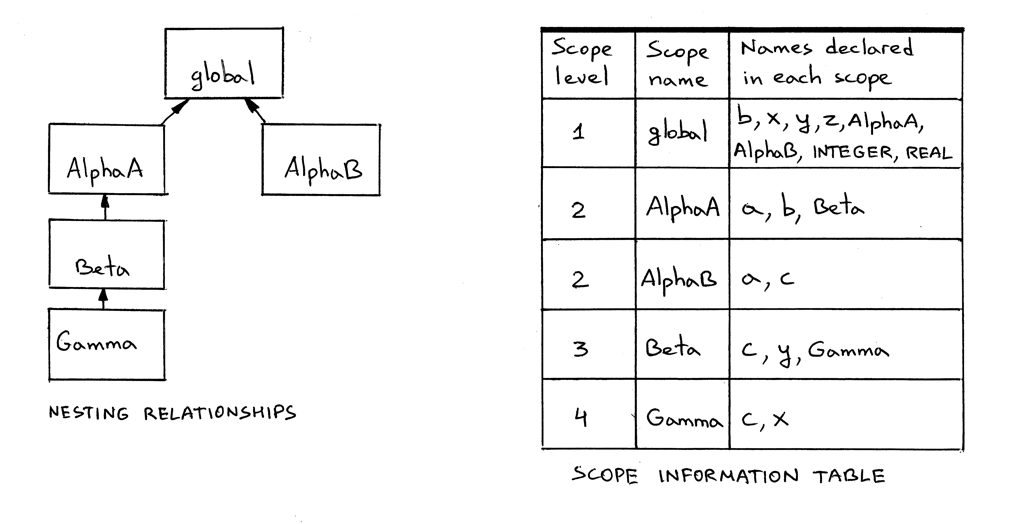Let’s run our source-to-source compiler and inspect the output. The subscripts should match the ones in the scope information table in the picture above:

```\$ python src2srccompiler.py nestedscopes04.pas
program Main0;
var b1 : REAL;
var x1 : REAL;
var y1 : REAL;
var z1 : INTEGER;
procedure AlphaA1(a2 : INTEGER);
var b2 : INTEGER;
procedure Beta2(c3 : INTEGER);
var y3 : INTEGER;
procedure Gamma3(c4 : INTEGER);
var x4 : INTEGER;

begin
<x4:INTEGER> := <a2:INTEGER> + <b2:INTEGER> + <c4:INTEGER> + <x4:INTEGER> + <y3:INTEGER> + <z1:INTEGER>;
end; {END OF Gamma}

begin

end; {END OF Beta}

begin

end; {END OF AlphaA}
procedure AlphaB1(a2 : INTEGER);
var c2 : REAL;

begin
<c2:REAL> := <a2:INTEGER> + <b1:REAL>;
end; {END OF AlphaB}

begin

end. {END OF Main}
```

Spend some time studying both the pictures and the output of the source-to-source compiler. Make sure you understand the following main points:

• The way the vertical lines are drawn to show the scope of the declarations.
• That a hole in a scope indicates that a variable is re-declared in a nested scope.
• That AlphaA and AlphaB are declared in the global scope.
• That AlphaA and AlphaB declarations introduce new scopes.
• How scopes are nested within each other, and their nesting relationships.
• Why different names, including variable references in assignment statements, are subscripted the way they are. In other words, how name resolution and specifically the lookup method of chained scoped symbol tables works.

Also run the following program:

```\$ python scope05.py nestedscopes04.pas
```

and inspect the contents of the chained scoped symbol tables and compare it with what you see in the scope information table in the picture above. And don’t forget about the genastdot.py, which you can use to generate a visual diagram of an AST to see how procedures are nested within each other in the tree.

Before we wrap up our discussion of nested scopes for today, recall that earlier we removed the semantic check that was checking source programs for duplicate identifiers. Let’s put it back. For the check to work in the presence of nested scopes and the new behavior of the lookup method, though, we need to make some changes. First, we need to update the lookup method and add an extra parameter that will allow us to limit our search to the current scope only:

```def lookup(self, name, current_scope_only=False):
print('Lookup: %s. (Scope name: %s)' % (name, self.scope_name))
# 'symbol' is either an instance of the Symbol class or None
symbol = self._symbols.get(name)

if symbol is not None:
return symbol

if current_scope_only:
return None

# recursively go up the chain and lookup the name
if self.enclosing_scope is not None:
return self.enclosing_scope.lookup(name)
```

And second, we need to modify the visit_VarDecl method and add the check using our new current_scope_only parameter in the lookup method:

```def visit_VarDecl(self, node):
type_name = node.type_node.value
type_symbol = self.current_scope.lookup(type_name)

# We have all the information we need to create a variable symbol.
# Create the symbol and insert it into the symbol table.
var_name = node.var_node.value
var_symbol = VarSymbol(var_name, type_symbol)

# Signal an error if the table alrady has a symbol
# with the same name
if self.current_scope.lookup(var_name, current_scope_only=True):
raise Exception(
"Error: Duplicate identifier '%s' found" % var_name
)

self.current_scope.insert(var_symbol)
```

If we don’t limit the search for a duplicate identifier to the current scope, the lookup might find a variable symbol with the same name in an outer scope and, as a result, would throw an error, while in reality there was no semantic error to begin with.

Here is the output from running scope05.py with a program that doesn’t have duplicate identifier errors. You can notice below that the output has more lines in it, due to our duplicate identifier check that looks up for a duplicate name before inserting a new symbol:

```\$ python scope05.py nestedscopes02.pas
ENTER scope: global
Insert: INTEGER
Insert: REAL
Lookup: REAL. (Scope name: global)
Lookup: x. (Scope name: global)
Insert: x
Lookup: REAL. (Scope name: global)
Lookup: y. (Scope name: global)
Insert: y
Insert: Alpha
ENTER scope: Alpha
Lookup: INTEGER. (Scope name: Alpha)
Lookup: INTEGER. (Scope name: global)
Insert: a
Lookup: INTEGER. (Scope name: Alpha)
Lookup: INTEGER. (Scope name: global)
Lookup: y. (Scope name: Alpha)
Insert: y
Lookup: a. (Scope name: Alpha)
Lookup: x. (Scope name: Alpha)
Lookup: x. (Scope name: global)
Lookup: y. (Scope name: Alpha)
Lookup: x. (Scope name: Alpha)
Lookup: x. (Scope name: global)

SCOPE (SCOPED SYMBOL TABLE)
===========================
Scope name     : Alpha
Scope level    : 2
Enclosing scope: global
Scope (Scoped symbol table) contents
------------------------------------
a: <VarSymbol(name='a', type='INTEGER')>
y: <VarSymbol(name='y', type='INTEGER')>

LEAVE scope: Alpha

SCOPE (SCOPED SYMBOL TABLE)
===========================
Scope name     : global
Scope level    : 1
Enclosing scope: None
Scope (Scoped symbol table) contents
------------------------------------
INTEGER: <BuiltinTypeSymbol(name='INTEGER')>
REAL: <BuiltinTypeSymbol(name='REAL')>
x: <VarSymbol(name='x', type='REAL')>
y: <VarSymbol(name='y', type='REAL')>
Alpha: <ProcedureSymbol(name=Alpha, parameters=[<VarSymbol(name='a', type='INTEGER')>])>

LEAVE scope: global
```

Now, let’s take scope05.py for another test drive and see how it catches a duplicate identifier semantic error.

For example, for the following erroneous program with a duplicate declaration of a in the Alpha scope:

```program Main;
var x, y: real;

procedure Alpha(a : integer);
var y : integer;
var a : real;  { ERROR here! }
begin
x := a + x + y;
end;

begin { Main }

end.  { Main }
```

the program generates the following output:

```\$ python scope05.py dupiderror.pas
ENTER scope: global
Insert: INTEGER
Insert: REAL
Lookup: REAL. (Scope name: global)
Lookup: x. (Scope name: global)
Insert: x
Lookup: REAL. (Scope name: global)
Lookup: y. (Scope name: global)
Insert: y
Insert: Alpha
ENTER scope: Alpha
Lookup: INTEGER. (Scope name: Alpha)
Lookup: INTEGER. (Scope name: global)
Insert: a
Lookup: INTEGER. (Scope name: Alpha)
Lookup: INTEGER. (Scope name: global)
Lookup: y. (Scope name: Alpha)
Insert: y
Lookup: REAL. (Scope name: Alpha)
Lookup: REAL. (Scope name: global)
Lookup: a. (Scope name: Alpha)
Error: Duplicate identifier 'a' found
```

It caught the error as expected.

On this positive note, let’s wrap up our discussion of scopes, scoped symbol tables, and nested scopes for today.

### Summary

We’ve covered a lot of ground. Let’s quickly recap what we learned in this article:

• We learned about scopes, why they are useful, and how to implement them in code.
• We learned about nested scopes and how chained scoped symbol tables are used to implement nested scopes.
• We learned how to code a semantic analyzer that walks an AST, builds scoped symbols tables, chains them together, and does various semantic checks.
• We learned about name resolution and how the semantic analyzer resolves names to their declarations using chained scoped symbol tables (scopes) and how the lookup method recursively goes up the chain in a scope tree to find a declaration corresponding to a certain name.
• We learned that building a scope tree in the semantic analyzer involves walking an AST, “pushing” a new scope on top of a scoped symbol table stack when ENTERing a certain AST node and “popping” the scope off the stack when LEAVing the node, making a scope tree look like a collection of scoped symbol table stacks.
• We learned how to write a source-to-source compiler, which can be a useful tool when learning about nested scopes, scope levels, and name resolution.

### Exercises

Time for exercises, oh yeah!1. You’ve seen in the pictures throughout the article that the Main name in a program statement had subscript zero. I also mentioned that the program’s name is not in the global scope and it’s in some other outer scope that has level zero. Extend spi.py and create a builtins scope, a new scope at level 0, and move the built-in types INTEGER and REAL into that scope. For fun and practice, you can also update the code to put the program name into that scope as well.

2. For the source program in nestedscopes04.pas do the following:

1. Write down the source Pascal program on a piece of paper
2. Subscript every name in the program indicating the scope level of the declaration the name resolves to.
3. Draw vertical lines for every name declaration (variable and procedure) to visually show its scope. Don’t forget about scope holes and their meaning when drawing.
4. Write a source-to-source compiler for the program without looking at the example source-to-source compiler in this article.
5. Use the original src2srccompiler.py program to verify the output from your compiler and whether you subscripted the names correctly in the exercise (2.2).
3. Modify the source-to-source compiler to add subscripts to the built-in types INTEGER and REAL

4. Uncomment the following block in the spi.py

```# interpreter = Interpreter(tree)
# result = interpreter.interpret()
# print('')
# print('Run-time GLOBAL_MEMORY contents:')
# for k, v in sorted(interpreter.GLOBAL_MEMORY.items()):
#     print('%s = %s' % (k, v))
```

Run the interpreter with the part10.pas file as an input:

```\$ python spi.py part10.pas
```

Spot the problems and add the missing methods to the semantic analyzer.

That’s it for today. In the next article we’ll learn about runtime, call stack, implement procedure calls, and write our first version of a recursive factorial function. Stay tuned and see you soon!

If you’re interested, here is a list of books (affiliate links) I referred to most when preparing the article:

1. Language Implementation Patterns: Create Your Own Domain-Specific and General Programming Languages (Pragmatic Programmers)2. Engineering a Compiler, Second Edition3. Programming Language Pragmatics, Fourth Edition4. Compilers: Principles, Techniques, and Tools (2nd Edition)5. Writing Compilers and Interpreters: A Software Engineering Approach